• Home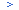• Support• FAQs & Downloads• Timepieces• Watch with slide rule# Watch with slide rule

Q1 How is the slide rule used?
A1
 A. General Calculation Functions 1) Multiplication[Example]12 x 15 [Operation]Align 12 on the outer scale with 10 on the inner scale.Then, 15 on the inner scale corresponds to 18 on the outer scale.Take into account the position of the decimal point and add one zero toobtain 180. Note that with the scales of this watch, the position of thedecimal point cannot be obtained.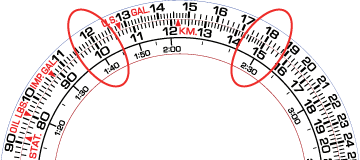2) Division [Example]300 / 15 [Operation]Align 30 on the outer scale with 15 on the inner scale.Then, 10 on the inner scale corresponds to 20 on the outer scale.Take into account the position of the decimal point to obtain 20.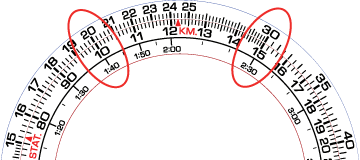3) Reading Ratios [Example]50 / 20 = 30 / x [Operation]Align 50 on the outer scale with 20 on the inner scale.Then, 30 on the outer scale corresponds to 12 on the inner scale.At this point, the proportion for every value on the inner and outerscales is "50:20".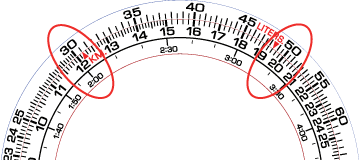4) Determining Square Root [Example]Square root of 169 [Operation]Turn the outer scale slowly and find a value that corresponds to both 16.9 on the outer scale and 10 on the inner scale.In this example, 16.9 on the outer scale corresponds to 13 onthe inner scale, and 10 on the inner scale corresponds to 13on the outer scale. Thus, the answer is 13.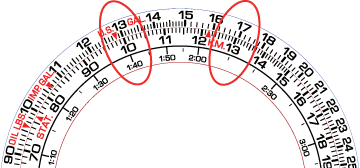B. Navigation Calculator for motor sports 1) Time required [Example]Obtain the time required 55 kilometers per hour for 330 kilometers. [Operation]Align 55 on the outer scale with the SPEED INDEX (MPH) on the inner scale.Then, 33 on the outer scale corresponds to "6:00" on the innerscale (time scale).Thus, the time required is 6 hours.* It is calculable also as mile instead of kilometer.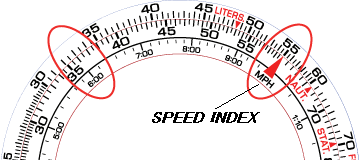2) Speed [Example]Obtain the kilometers per hour for 120 kilometers with a time of1 hour and 30 minutes. [Operation]Align 12 on the outer scale with "1:30" on the inner scale (time scale).Then, the SPEED INDEX (MPH) on the inner scale corresponds to 80 on theouter scale. Thus, the speed is 80 kilometers per hour.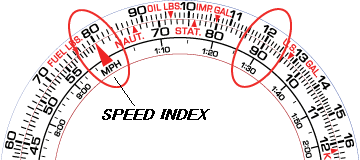3) Mileage [Example]Obtain the mileage when the speed is 40 kilometers per hour and the runningtime is 1 hour and 30 minutes. [Operation]Align 40 on the outer scale with the SPEED INDEX (MPH) on the inner scale.Then, "1:30" on the inner scale (time scale) corresponds to 60 on theouter scale. Thus, the mileage is 60 kilometers.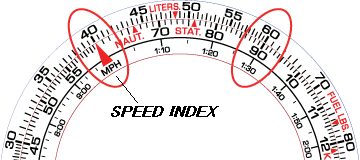4) Rate of fuel consumption [Example]Obtain the rate of fuel consumption (liters / hour) when the running timeis 5 hours and the fuel consumption is 35 liters. [Operation]Align 35 on the outer scale with "5:00" on the inner scale (time scale).Then, the SPEED INDEX (MPH) on the inner scale corresponds to 70 on theouter scale. Thus, the fuel consumption is 7 liters per hour.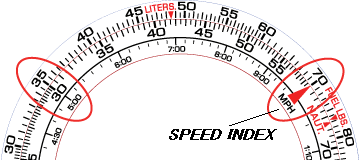5) Fuel consumption [Example]Obtain the fuel consumption required for a running when the rate of fuelconsumption is 6 liters per hour and the running time is 5 hours. [Operation]Align 60 on the outer scale with the SPEED INDEX (MPH) on the inner scale.Then, "5:00" on the inner scale (time scale) corresponds to 30 on theouter scale. Thus, the fuel consumption is 30 liters.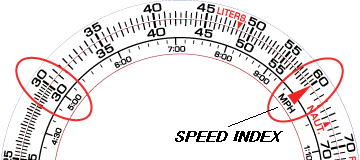6) Estimated running time [Example]Obtain the estimated running time when the rate of fuel consumption is5 liters per hour and the running has 30 liters of fuel. [Operation]Align 50 on the outer scale with the SPEED INDEX (MPH) on the inner scale.Then, 30 on the outer scale corresponds to "6:00" on the inner scale (time scale).Thus, the estimated running time is 6 hours.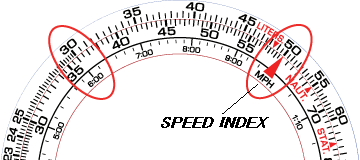C. Navigation Calculator for sky sports 1) Time required [Example]Obtain the time required for the flight of an aircraft at 160 knots for 240nautical miles. [Operation]Align 16 on the outer scale with the SPEED INDEX (MPH) on the inner scale.Then, 24 on the outer scale corresponds to "1:30" on the inner scale (time scale). Thus, the time required for the flight is 1 hours and 30 minutes.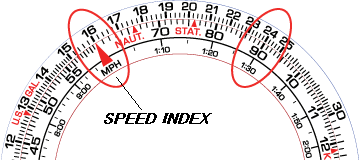2) Speed [Example]Obtain the knots (air speed) for 250 nautical miles with a flight time of1 hour and 40 minutes. [Operation]Align 25 on the outer scale with "1:40" on the inner scale (time scale).Then, the SPEED INDEX (MPH) on the inner scale corresponds to 15 on theouter scale. Thus, the air speed for the flight is 150 knots.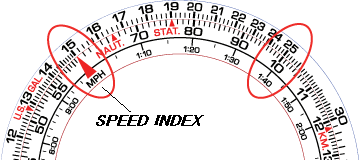3) Flight distance [Example]Obtain the air distance when the air speed is 180 knots and the flighttime is 40 minutes. [Operation]Align 18 on the outer scale with the SPEED INDEX (MPH) on the inner scale.Then, 40 on the inner scale corresponds to 12 on the outer scale.Thus, the air distance of the flight is 120 nautical miles.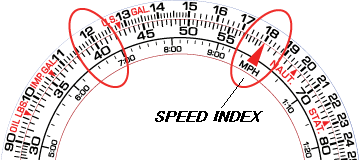4) Rate of fuel consumption [Example]Obtain the rate of fuel consumption (gallons / hour) when the flighttime is 40 minutes and the fuel consumption is 140 gallons. [Operation]Align 14 on the outer scale with 40 on the inner scale. Then, theSPEED INDEX (MPH) on the inner scale corresponds to 21 on the outer scale.Thus, the fuel consumption is 210 gallons per hour.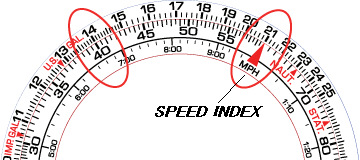5) Fuel consumption [Example]Obtain the fuel consumption required for a flight when the rate of fuel consumptionis 240 gallons per hour and the flight time is 1 hours and 40 minutes. [Operation]Align 24 on the outer scale with the SPEED INDEX (MPH) on the inner scale.Then, "1:40" on the inner scale (time scale) corresponds to 40 on theouter scale. Thus, the fuel consumption is 400 gallons.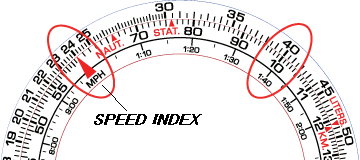6) Estimated flight time [Example]Obtain the estimated flight time when the rate of fuel consumption is 200 gallonsper hour and the aircraft has 1400 gallons of fuel. [Operation]Align 20 on the outer scale with the SPEED INDEX (MPH) on the inner scale.Then, 14 on the outer scale corresponds to "7:00" on the inner scale (time scale).Thus, the estimated flight time is 7 hours.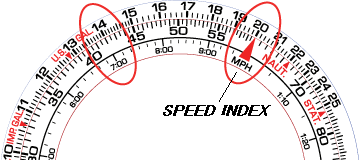D. Conversion 1) Distance [Example]Convert 45 miles into nautical miles and kilometers. [Operation]Align 45 on the outer scale with STAT on the inner scale.Then, NAUT on the inner scale corresponds to about 39 nautical mileson the outer scale, and KM on the inner scale corresponds to about72 km on the outer scale.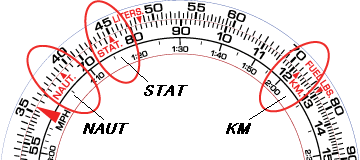2) Weight [Example]Convert 16.4 oil lbs. into U.S. gallons and IMP. gallons and liters. [Operation]Align 16.4 on the inner scale with OIL LBS on the outer scale.Then, U.S. GAL on the outer scale corresponds to about 2.2 U.S. gallonson the inner scale, and IMP. GAL on the outer scale corresponds to about1.8 IMP. gallons on the inner scale, and LITERS on the outer scalecorresponds to about 8.3 liters on the inner scale.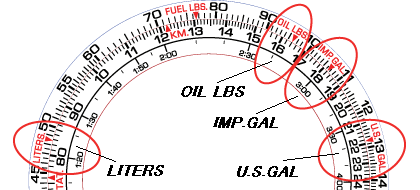3) Volume [Example]Convert 13.1 fuel lbs. into U.S. gallons and IMP. gallons and liters. [Operation]Align 13.1 on the inner scale with FUEL LBS on the outer scale.Then, U.S. GAL on the outer scale corresponds to about 2.2 U.S. gallonson the inner scale, and IMP. GAL on the outer scale corresponds to about1.8 IMP. gallons on the inner scale, and LITERS on the outer scalecorresponds to about 8.3 liters on the inner scale.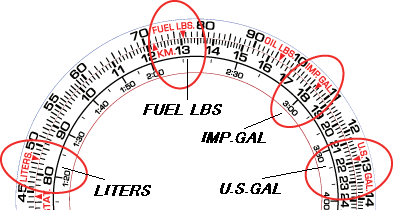4) Fuel [Example]Convert 16.8 U.S. gallons into and IMP. gallons and liters. [Operation]Align 16.8 on the inner scale with U.S. GAL on the outer scale.Then, IMP. GAL on the outer scale corresponds to about 14 IMP. gallonson the inner scale, and LITERS on the outer scale corresponds to about63.5 liters on the inner scale.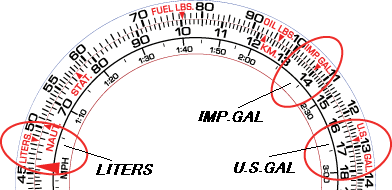How useful was this information? Excellent Good Fair Poor